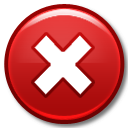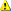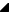﻿ TRSChartValue.ValueCount Property# TRSChartValue.ValueCount Property

Returns the number of dimensions in the chart value. A chart value, depending on the chart type, can contain 1 or more dimensional values that define the chart value.

For example, each chart value in a bar chart contains 1 value (defining the height of the bar), line chart values contains 2 dimensions: X and Y, and shape chart values contain 4 dimensions: X, Y, Width, and Height.

Use the Values property to access each dimension in the chart value. The Value property in comparison returns the main (or only) dimension in a chart value - which dimension is returned is based on the chart type.Caution

Note that TRSChartValue descendant classes expose properties, such as X and Y, which are safer to access the specific dimension of the value. You should not count on a specific dimension index to contain a specific property

Namespace: RSChartPanelSyntax
 Delphi public   property ValueCount: Integer read GetValueCount;

Property Value

Type: IntegerExamples

The following code builds a string containing the Caption for the value and all of the floating point numbers contained in the TRSChartValue:

Delphi

var
i: Integer;
begin
result := Caption + ' = ';
for i := 0 to ValueCount - 1 do
begin
result := result + FormatFloat( Fmt, Values[i] );
if i < (ValueCount - 1) then
result := result + ', ';
end;
end;See Also

#### Reference

 • RSChartPanel.TRSChartValue.Value
 • TRSChartValue
 • RSChartPanel

RiverSoftAVG Charting Component Suite (RCCS) © 2005-2015, Thomas G. Grubb